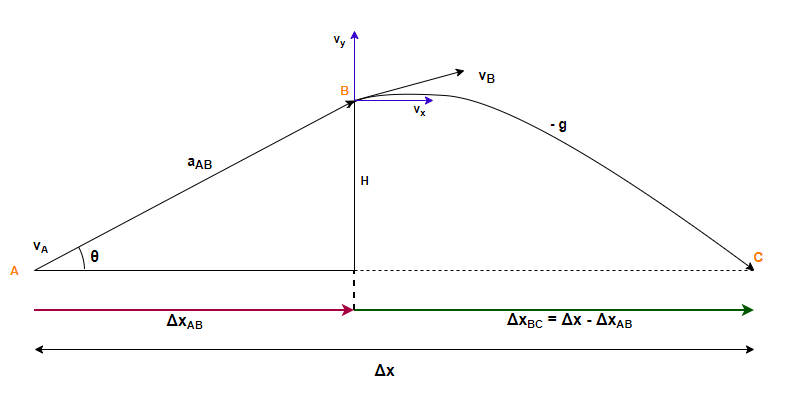# Problem: A skateboarder starts up a 1.0-m-high, 30° ramp at a speed of 7.1 m/s. The skateboard wheels roll without friction. At the top, she leaves the ramp and sails through the air.How far from the end of the ramp does the skateboarder touch down?

###### FREE Expert Solution

In this problem, we're asked to determine displacement from the end of the ramp.

This is a Positive Launch From Height Up Ramp type of a problem. We'll follow these steps:

1.  Draw diagram and label axes.
2. Choose a UAM equation—sometimes you'll be able to go directly for the target variable, sometimes another step will be needed in between. Identify the target and known variables for each direction.
3. Solve the equation for the target (or intermediate) variable, then substitute known values and calculate the answer.

Our problem has motion in both x- and y-directions.

The four UAM (kinematics) equations for motion in the x-direction are:

For motion in the y-direction, the UAM equations will be:

For a positive launch angle, we also need to know how to decompose a velocity vector into its x- and y-components:

Step 1: Draw diagram and label axes

This motion has two separate parts. In interval AB, all the motion is parallel to the ramp. That means we can treat this part of the motion as one-dimensional instead of decomposing any of the vectors.

In interval BC, we have projectile motion. The skateboarder’s launch velocity for this motion is vB. If we can calculate vB from the givens for interval AB, the rest of the problem will be exactly like any other projectile motion problem with a positive launch angle: we’ll just replace v0 with vB.In our coordinate system, the +y-axis is pointing upwards and the +x-direction is horizontal along the launch direction.

79% (215 ratings)###### Problem Details

A skateboarder starts up a 1.0-m-high, 30° ramp at a speed of 7.1 m/s. The skateboard wheels roll without friction. At the top, she leaves the ramp and sails through the air.

How far from the end of the ramp does the skateboarder touch down?